Anass AI Scientist & PhD Candidate. Talking Science, Philosophy and Technology.

# Improve your Visualization Design on PyhtonIt is impossible to know everything, no matter how much our experience has increased over the years, there are many things that remain hidden from us. This is normal, and maybe an exciting motivation to search and learn more. And I am sure that this is what drove you to this article.

We know that one of Matplotlib’s most important features is its ability to play well with many operating systems and graphics backends. Matplotlib supports dozens of backends and output types, which means you can count on it to work regardless of which operating system you are using or which output format you wish .

I am sharing with you 5 magical tricks and new features I didn’t know about before, to improve your design and visualization skills using Matplotlib. These tricks will lend a helping hand to your work and make it more professional.

In case one of these features did not work for you, please update your Matplotlib version using:

``````1
pip install -U matplotlib
``````

Without further ado, let’s get started!

## Trick 1 : Plots Annotation

Our first trick for today is annotations which are types of comments added to a plot at a point to make it more understandable, clarify more information, or define the role of that point.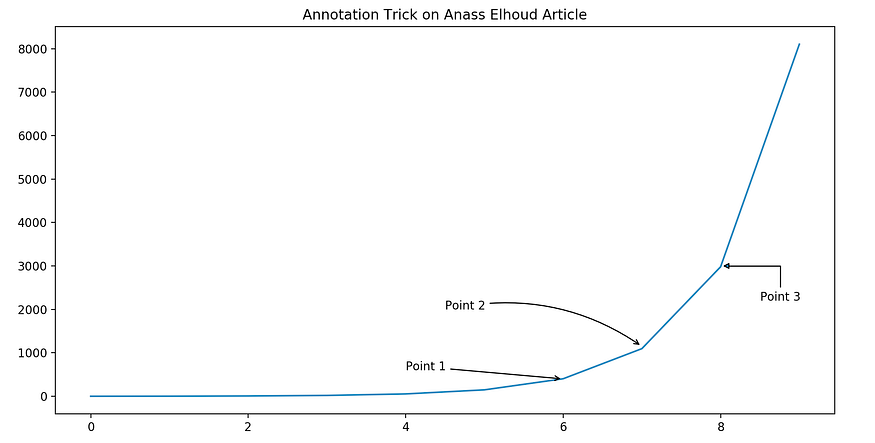To do so, we are going to use plt.annotate() function from Matplotlib. It allows you to create arrows, join them, and make them point to a specific zone. You can adapt the lines above to your own code:

``````1
2
3
4
5
6
7
8
9
10
11
12
13
14
15
16
import matplotlib.pyplot as plt
import numpy as np
import pandas as pd
import random

X=list(range(10))
Y=[x+(x*random.random()) for x in X]

fig=plt.figure(figsize=(12,6))

plt.plot(X,np.exp(X))
plt.title('Annotation Trick on Anass Elhoud Article')
plt.annotate('Point 1',xy=(6,400),arrowprops=dict(arrowstyle='->'),xytext=(4,600))
plt.annotate('Point 3',xy=(8,3000),arrowprops=dict(arrowstyle='-|>',connectionstyle='angle,angleA=90,angleB=0'),xytext=(8.5,2200))
plt.show()
``````

This method will definitely help you to present your work either in writing, in a Latex report, Ph.D. defense, and so on…

## Trick 2 : Zoom Method

I think this is the most magical trick to mention. This new feature is so helpful and interesting, especially for researchers and data scientists. The method indicate_inset_zoom() returns a rectangle showing where the zoom is located which helps you show a specific part of the curve without plotting another one.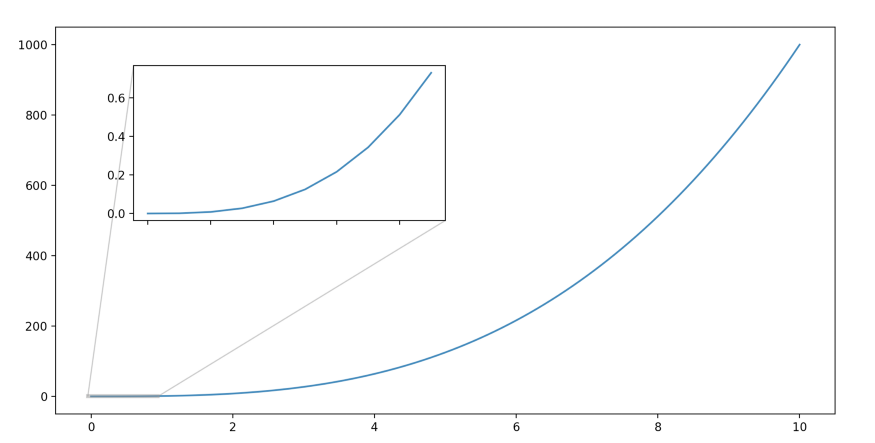The code above explains how to call the method and add it to your plot:

``````1
2
3
4
5
6
7
8
9
10
11
12
step=.1
x=np.arange(0,10+step,step)
y=x**3

fig,ax=plt.subplots(figsize=(12,6))
ax.plot(x,y)
axins=ax.inset_axes([0.1,0.5,0.4,0.4])
axins.plot(x[:10],y[:10])
ax.indicate_inset_zoom(axins,linewidth=3)
axins.set_xticklabels('')
axins.set_xticklabels('')
plt.show()
``````

## Trick 3 : Watermark on the plot

This trick is useful for solving copyrights issues. It helps you add your watermark to your visualization design. It is not very widely used but it is still an important feature to know about when preparing your visualization project. In this feature, you can either use a text watermark or an image watermark.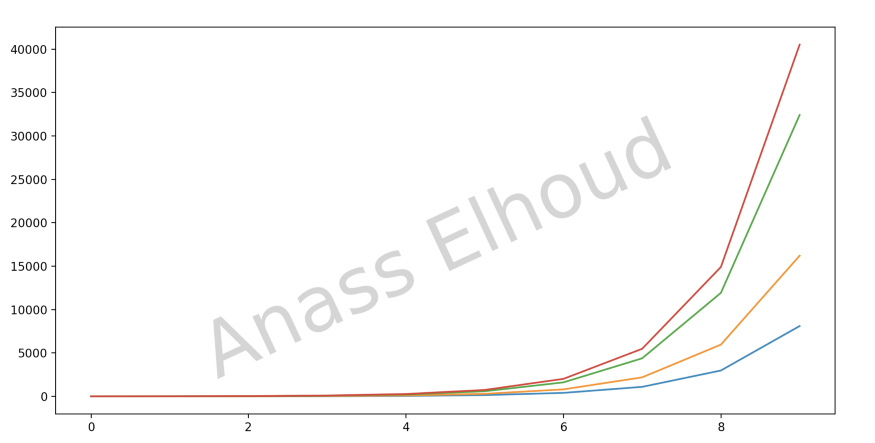To add a text watermark, you can use the line below:

``````1
2
3
4
5
6
7
8
9
10
11
12
13
14
15
X=list(range(10))
Y=[x+(x*random.random()) for x in X]

fig=plt.figure(figsize=(12,6))

plt.plot(X,np.exp(X))
plt.plot(X,2*np.exp(X))
plt.plot(X,4*np.exp(X))
plt.plot(X,5*np.exp(X))
# Text Watermark
fig.text(0.75,0.15, 'Anass Elhoud',
fontsize=65, color='gray',
ha='right', va='bottom', alpha=0.4,rotation=25)
plt.legend(loc='upper center',ncol=2,frameon=False)
plt.show()
``````

You can also use your logo or your company’s logo as a watermark by changing the lines 11, 12, and 13 on the code above.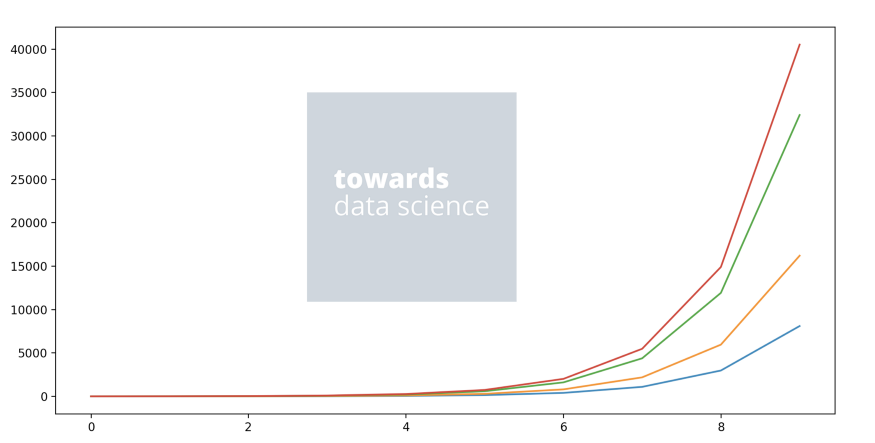Once you added your logo to the same directory as your main file, you can use this code instead of the text watermark. Do not forget to modify “tds.png” by your own logo name:

``````1
2
3
4
5
import matplotlib.image as img

fig.figimage(av_logo,900,400,alpha=0.3)
plt.show()
``````

## Trick 4: Sharing axes

These new methods allow sharing axes immediately after creating them. Which gives an attractive and arranged look to your visualizations. Please make sure you update your Matplotlib version before using the code because this method is a newly published feature.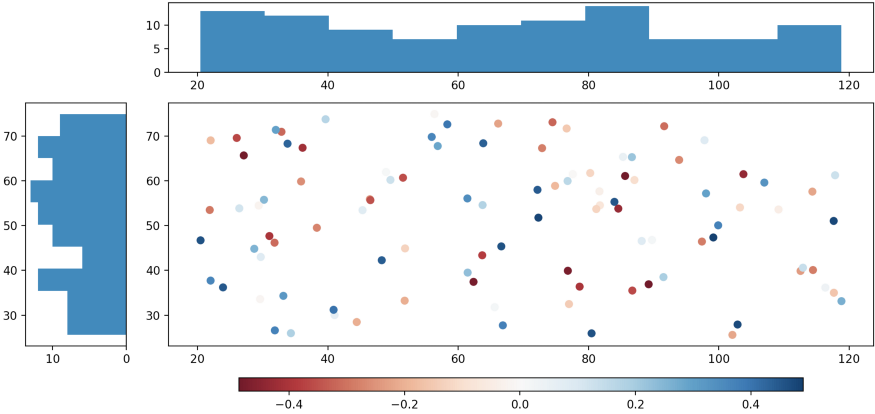The code source is written below:

``````1
2
3
4
5
6
7
8
9
10
11
12
13
14
15
16
17
18
19
np.random.seed(0)
x = np.random.random(100) * 100 + 20
y = np.random.random(100) * 50 + 25
c = np.random.random(100) - 0.5

fig = plt.figure(constrained_layout=True)
axd = fig.subplot_mosaic([['.', 'histx'], ['histy', 'scat']],
gridspec_kw={'width_ratios': [1, 7],
'height_ratios': [2, 7]})

axd['histy'].invert_xaxis()
axd['histx'].sharex(axd['scat'])
axd['histy'].sharey(axd['scat'])

im = axd['scat'].scatter(x, y, c=c, cmap='RdBu', picker=True)
fig.colorbar(im, orientation='horizontal', ax=axd['scat'], shrink=0.8)
axd['histx'].hist(x)
axd['histy'].hist(y, orientation='horizontal')
plt.show()
``````

## Trick 5: Infinite lines

This trick is useful for Data Scientists and Machine Learning Engineers as well. It creates an infinite line passing through two points. It can be useful for separating clusters, groups, or plots.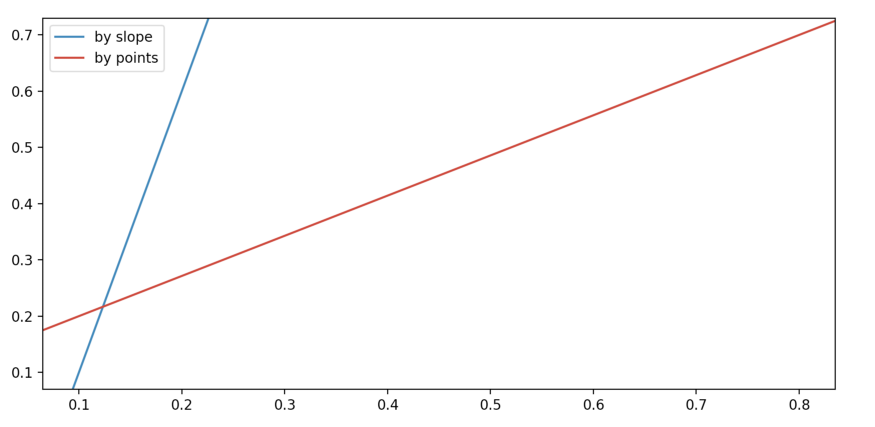Use the following code source to check this feature and let me see how would you apply it in your data visualization project:

``````1
2
3
4
5
6
7
fig, ax = plt.subplots()

ax.axline((.1, .1), slope=5, color='C0', label='by slope')
ax.axline((.1, .2), (.8, .7), color='C3', label='by points')

ax.legend()
plt.show()
``````

Data Scientist, ML Engineer, Data Analyst, or Business Analyst, you are surely aware of the power of Matplotlib and what is capable of. It is one of the best tools that help us tell stories efficiently and powerfully, linking our analysis to business objectives and get interpretation and decisions as results.

With these features, you will be able to add both elegance and professionalism to your academic or official projects. And you will make it easier for the readers and reviewers to understand and keep track of the information and the interpretation resulted from the data visualization.

There is just something extraordinary about a well-designed visualization: the colors stand out, the layers harmonize, the contours fit into the whole design, and it not only has a beautiful aesthetic quality but also gives us a meaningful insight. You know that data visualization is like telling a story, that’s why you should make it professional and enjoyable as much as you can.

Rating: